# Introduction

The goal of the project is to employ a machine learning model to infer gas composition (XH₂O and XCO₂) and temperature from a blended spectra including contributions from the two molecules. Obtaining such thermochemical properties of gas-phase systems, from simple diffusion flames to rocket engines, are critical for thoroughly understanding and identifying underlying complex combustion phenomena that effect overall combustion performance.

Laser absorption spectroscopy (LAS) is a diagnostic technique that is often well suited for combustion applications. LAS uses lasers to measure thermochemical properties in gases by absorption spectrometry. LAS is useful in circumstances requiring highly sensitive and selective measurements that are non-intrusive and cause no disturbance to the gas sample, as is often the case in combustion environments.

From wavelength-dependent light intensity absorbed by a gas, we can infer temperature and species concentrations from known molecular absorption spectral data. When a single species is present, this is often fairly straight forward by fitting individual spectral features for that molecule only. However, when many species are present, the spectra becomes blended with features from all molecules that absorb in that wavelength region and can become a complex non-linear problem that cannot be resolved using the typical line fitting processes; we refer to this as a convoluted spectra.

This project uses supervised machine learning as an alternative method for extracting temperature and mole fraction from a convoluted spectra of carbon monoxide (CO) and water (H₂O) by training with spectral data at known temperature and gas composition conditions.

# Dataset Creation

The molecular absorption spectral data used for training and testing are from HITRAN (High-resolution transmission molecular absorption database) which is open access. For several molecules, the database includes absorbance line positions, line strengths, and other spectral parameters from which absorbance at a given wavelength is obtained. The absorbance of a particular spectral feature is a function of temperature, pressure, and absorbing path length.

For this particular project, I downloaded an absorbance spectra dataset over a fixed frequency range (3770–3780 1/cm) at varied temperatures (1500–2500 K) and concentrations of CO₂ and H₂O (both from 0–10%). The spectral data includes 1000 conditions formulated by mesh grids of 10 equally spaced points for each variable over their respective range. From this greater dataset, the absorbance spectra at 100 conditions were set aside from training for the testing dataset. The test set was separated using the built-in sklearn train_test_split function.

Figure 1 shows the combined CO₂ and H₂O spectra at two randomly selected conditions from the dataset to illustrate the unique absorbance profiles over this frequency range affected by all of three of the varied parameters (T, XCO₂, XH₂O). Condition A represents spectra for a temperature of 1550 K, mole fraction of CO₂ of 9%, and mole fraction of water of 1% , while condition B represents spectra for a temperature of 2395 K, mole fraction of CO₂ of 3%, and mole fraction of water of 8%. This frequency range encompasses approximately 110 and 210 distant molecular absorbance lines of CO₂ and H₂O, respectively. Each of those lines has a unique temperature- and pressure-dependent spectral parameters that influence the strength and apparent shape of the feature. The absorbing path length was 10.3 cm, chosen to match that of the High Enthalpy Shock Tube (HEST) facility at UCLA.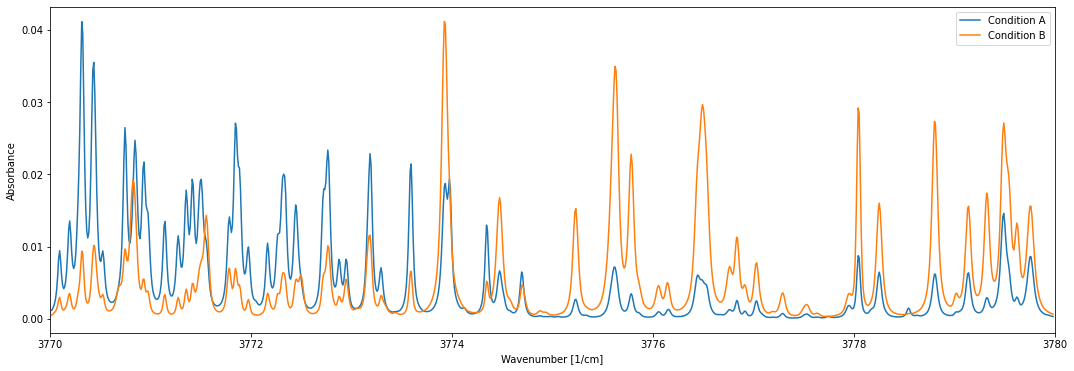Figure 1: CO and water convoluted absorbance spectra shown at two distinct temperature and gas composition conditions.

# Problem Formation and Model Selection

The input x data vector of the model is an absorbance vector comprised of the absorbance, v , at each wave number in the targeted range and the output y data vector contains the three thermodynamic properties (T, XCO, XHO)

To determine the best model for this application, several sklearn machine learning models were tested and their respective parameters varied in an attempt to minimize the root-mean-squared error (RMSE) and optimize the REC curve to reach the highest percentage of correct predictions achieved at a low window of error tolerance.

A simple linear regression model was used as a starting point to ensure the data loading and sorting was working as expected such that a decent model could be found. The three models tested thereafter include random forest, decision tree, and elastic net regression.

Following iterative attempts at optimizing parameters for each of the above models, the resulting RMSE values and REC curves, shown in Figure 2, the random forest model proved to be the most suitable for this work.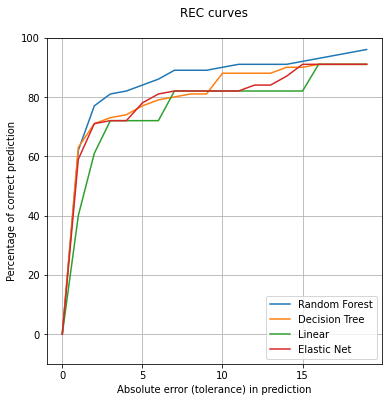Figure 2: REC curves for four tested regression models with optimized parameters.

# Random Forest Regression

Random forest regression involves supervised ensemble learning. In a random forest, it constructs multiple decision trees that run in parallel and do not influence each other and aggregates the results.

The finalized random forest regression model was setup with the following parameters: maximum features = 80, maximum depth = 70. When fit to the normalized spectral data, a RMSE of 0.17 and an out of bag R² of 0.96 were achieved, both of which were significant improvements compared to the other tested models.

Figure 3 plots the model predicted temperature and mole fractions versus the ground truth values for the test spectra. There is a clear linear correlation, as we expect to see for a correctly functioning model. The largest percentage error in prediction was consistently in the H₂O concentration values with an average error of 10% compared to 6 % for CO₂ and 5% for temperature.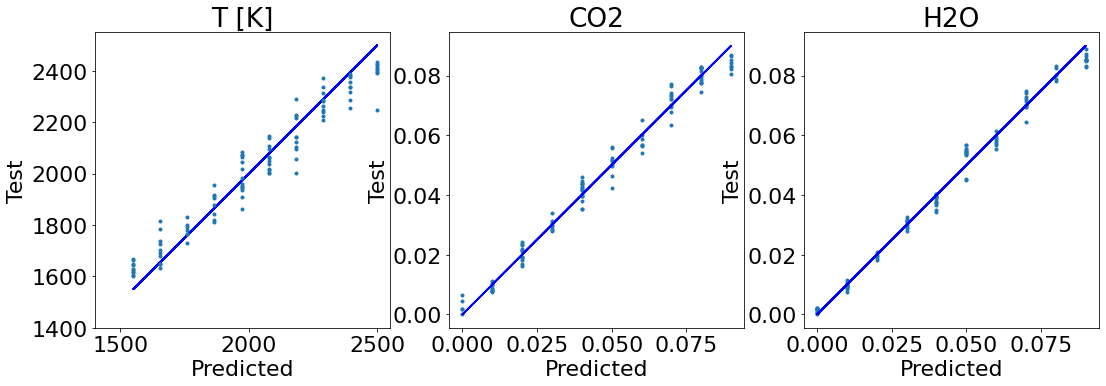Figure 3: Test data versus model predicted data shown for the three parameters: temperature, CO₂, and H₂O.

The 20 most ‘important’ features (wavenumber points) as determined by the model are presented in the histogram of Fig. 4. Of note, we see that many of these important wavenumbers lie within the line pair around 3775.7 1/cm which include absorbance transitions of H₂O with high temperature sensitivity.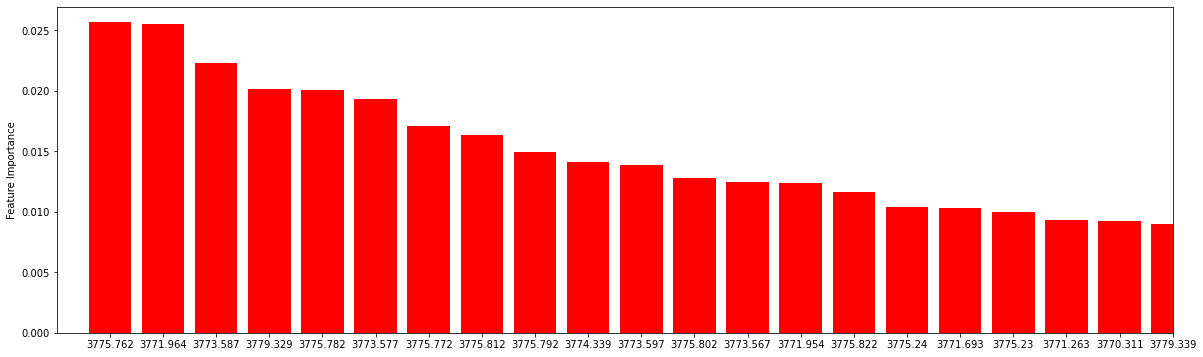Figure 4. Histogram of feature importance (labelled by wavenumber [cm^-1]) for the Random Forest model.

# Conclusion

In this work, a random forest machine learning model was successfully used to extract species mole fraction and temperature from convoluted absorption spectra of CO₂ and H₂O. This provides an exciting alternative to traditional spectral line fitting processes that are difficult to implement for complicated spectra. This model can be trained and used on data for these species in other wavelength regions as well as for other useful combustion species to measure.

--

--

--

Love podcasts or audiobooks? Learn on the go with our new app.

## How to Train SpaCy to Recognize Harry Potter Charms as Spell Entities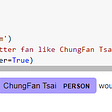## Assumptions in Linear Regression you might not know.## Using the latest advancements in deep learning to predict stock price movements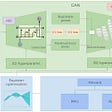## Introduction to Image Augmentations using the fastai library## Poor Man’s BERT — Why Pruning is Better than Knowledge Distillation ✂️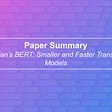## All you need to know about Google Vertex AI Matching Engine## What are the secret ingredients of Pinterest’s fashion recommendations? — Part 2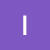## HIEA 122 Final Assignment## Is Technology bringing fourth a new era of tribalism?